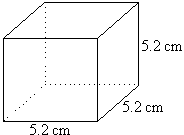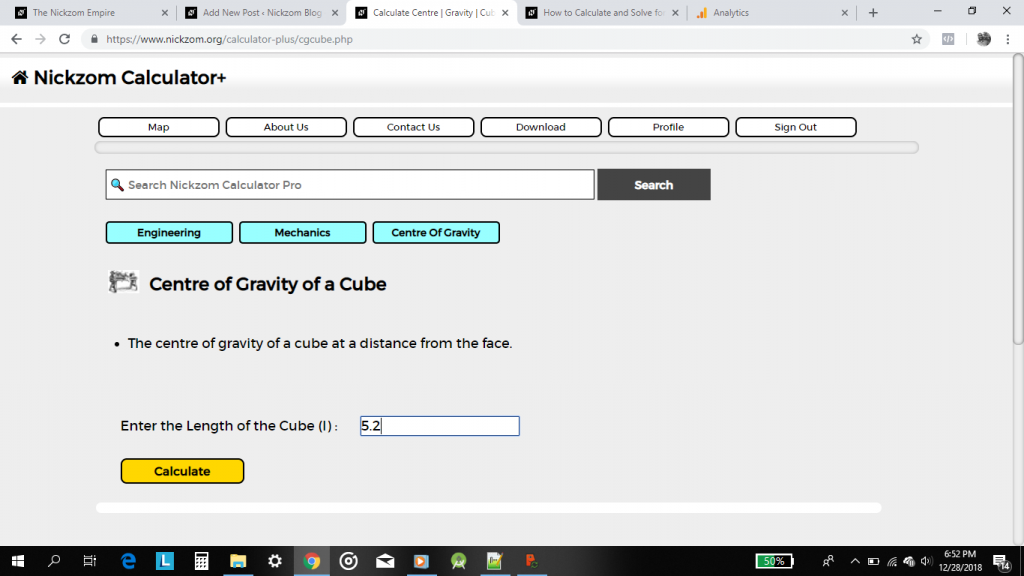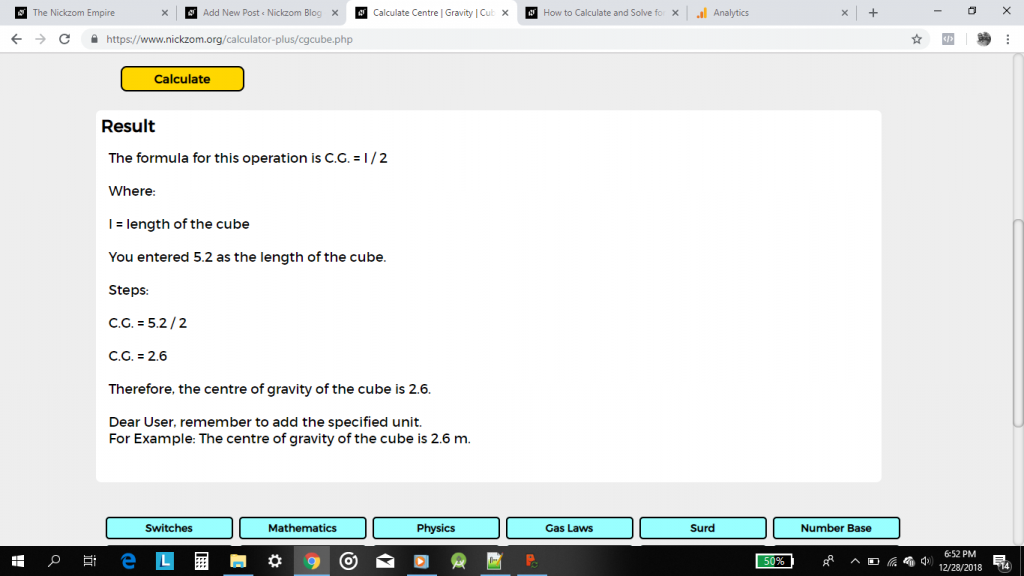# How to Calculate and Solve for the Centre of Gravity of a CubeThe image above is a cube with a length of 5.2 cm.
To compute the centre of gravity of a cube, one essential parameter is needed and this parameter is the length of the cube (l).

The formula for calculating the centre of gravity of a cube is:

C.G. = 0.5(l)

Where:
l = Length of the Cube
C.G. = Centre of Gravity

Let’s solve an example:
Find the centre of gravity of a cube where the length of a side of the cube is 5.2 cm.

This implies that:
l = Length of the Cube = 5.2

C.G. = 0.5(5.2)
C.G. = 2.6

Therefore, the centre of gravity of the cube is 2.6.

Nickzom Calculator – The Calculator Encyclopedia is capable of calculating the centre of gravity of a cube at a distance from the face.

To get the answer and workings of the center of gravity of a cube using the Nickzom Calculator – The Calculator Encyclopedia. First, you need to obtain the app.

You can get this app via any of these means:

To get access to the professional version via web, you need to register and subscribe for NGN 1,500 per annum to have utter access to all functionalities.
You can also try the demo version via https://www.nickzom.org/calculator

Once, you have obtained the calculator encyclopedia app, proceed to the Calculator Map, then click on Mechanics under the Engineering sectionNow, click on Centre of Gravity under StaticsNow, click on Centre of Gravity of a CubeThe screenshot below displays the page or activity to enter your value, to get the answer for the centre of gravity of a cube according to the respective parameter which is the length of the cube (l).Now, enter the value appropriately and accordingly for the parameter as required by the example above where the length of the cube is 5.2.Finally, click on CalculateAs you can see from the screenshot above, Nickzom Calculator – The Calculator Encyclopedia solves for the centre of gravity of a cube and presents the formula, workings and steps too.